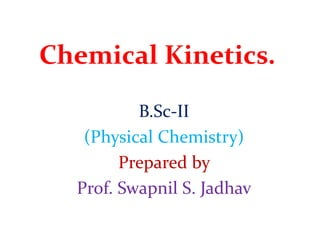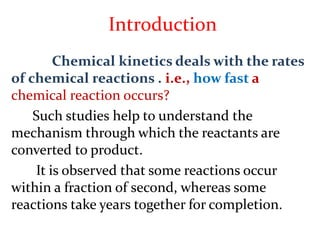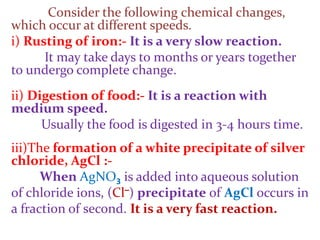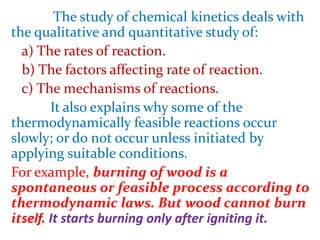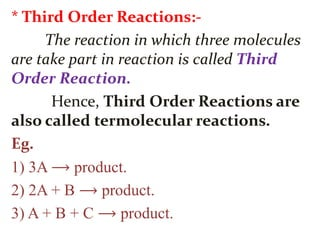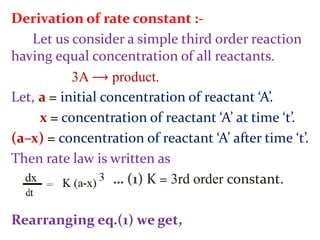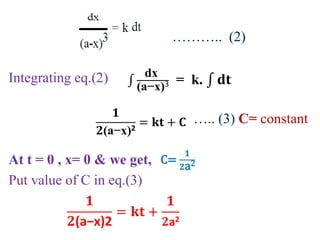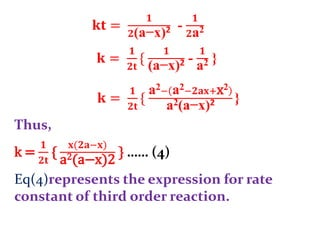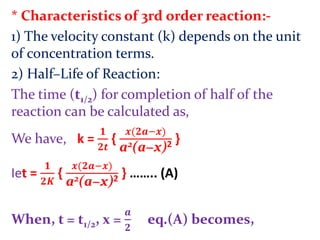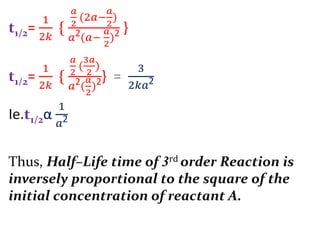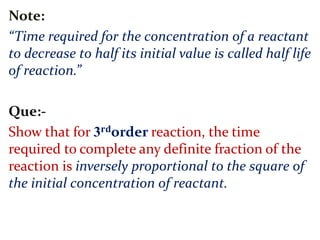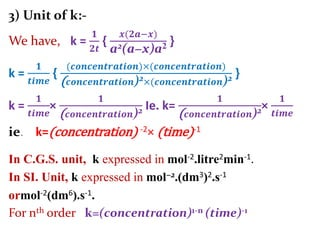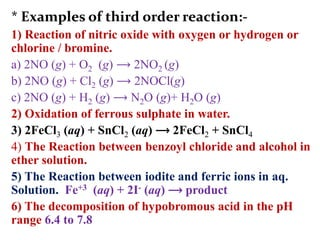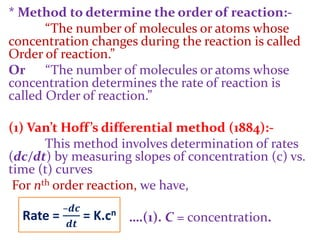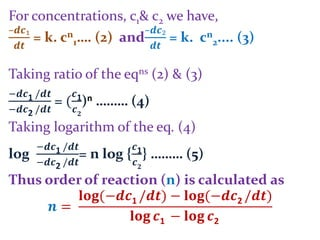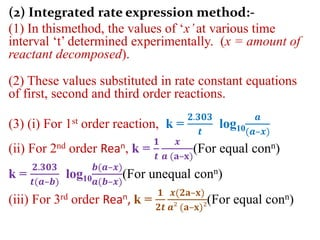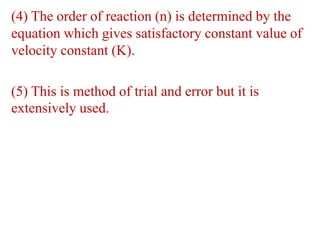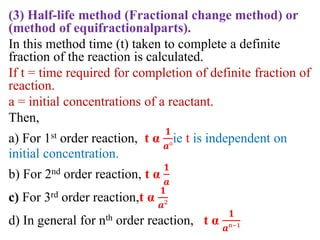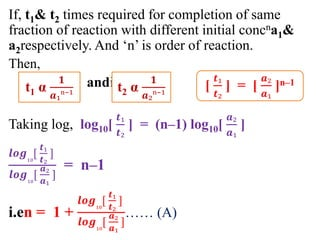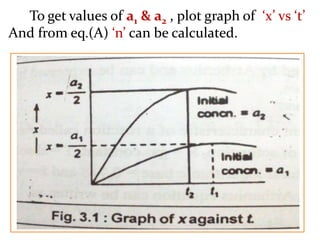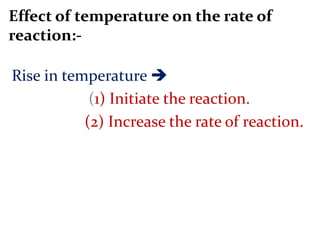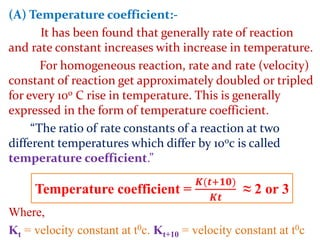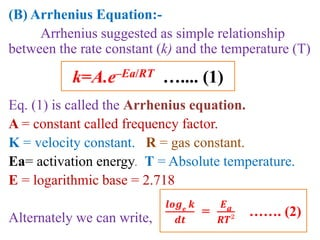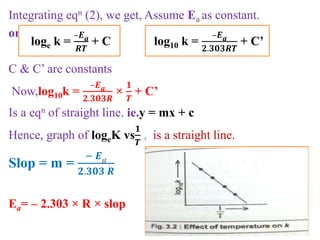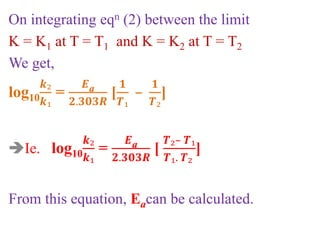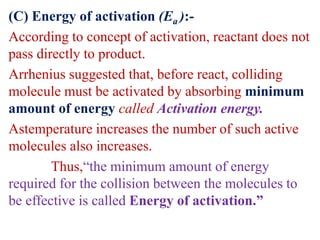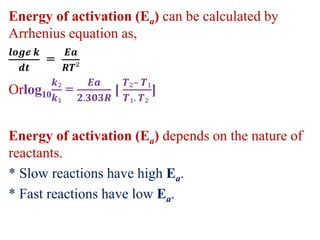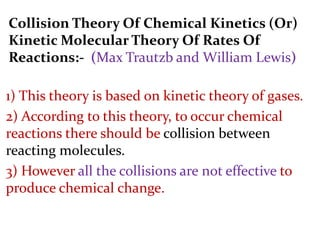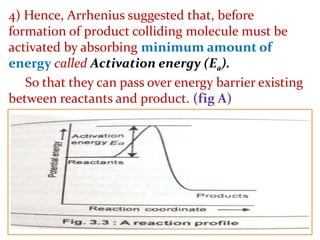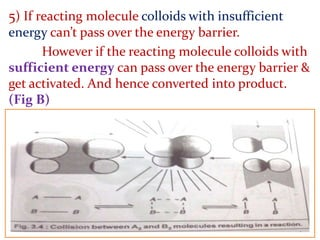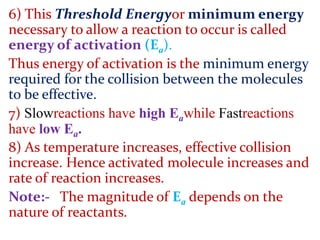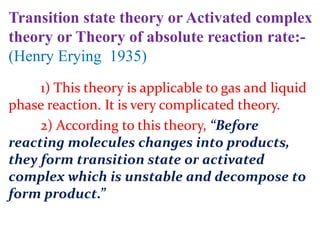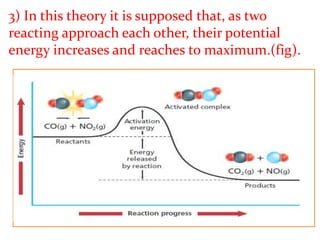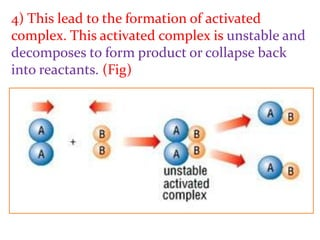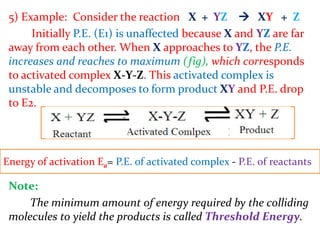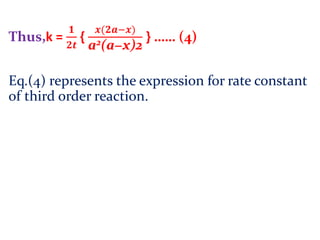1 sur 37

## RecommandéDifference between order and molecularity of a reaction 2310Prawin DdyChemical kineticsKendriya Vidyalaya SangathanOrder of reactionQURATULAIN MUGHALChem 2 - Chemical Kinetics VIII: The Arrhenius Equation, Activation Energy, a...Lumen LearningTypes of Organic ReactionsLiwayway Memije-CruzRoultes lawDanish Aakash

### Chemical Kinetics

1. Chemical Kinetics. B.Sc-II (Physical Chemistry) Prepared by Prof. Swapnil S. Jadhav
2. Introduction Chemical kinetics deals with the rates of chemical reactions . i.e., how fast a chemical reaction occurs? Such studies help to understand the mechanism through which the reactants are converted to product. It is observed that some reactions occur within a fraction of second, whereas some reactions take years together for completion.
3. Consider the following chemical changes, which occur at different speeds. i) Rusting of iron:- It is a very slow reaction. It may take days to months or years together to undergo complete change. ii) Digestion of food:- It is a reaction with medium speed. Usually the food is digested in 3-4 hours time. iii)The formation of a white precipitate of silver chloride, AgCl :- When AgNO3 is added into aqueous solution of chloride ions, (Cl–) precipitate of AgCl occurs in a fraction of second. It is a very fast reaction.
4. The study of chemical kinetics deals with the qualitative and quantitative study of: a) The rates of reaction. b) The factors affecting rate of reaction. c) The mechanisms of reactions. It also explains why some of the thermodynamically feasible reactions occur slowly; or do not occur unless initiated by applying suitable conditions. For example, burning of wood is a spontaneous or feasible process according to thermodynamic laws. But wood cannot burn itself. It starts burning only after igniting it.
5. * Third Order Reactions:- The reaction in which three molecules are take part in reaction is called Third Order Reaction. Hence, Third Order Reactions are also called termolecular reactions. Eg. 1) 3A ⟶ product. 2) 2A + B ⟶ product. 3) A + B + C ⟶ product.
6. Derivation of rate constant :- Let us consider a simple third order reaction having equal concentration of all reactants. 3A ⟶ product. Let, a = initial concentration of reactant ‘A’. x = concentration of reactant ‘A’ at time ‘t’. (a–x) = concentration of reactant ‘A’ after time ‘t’. Then rate law is written as … (1) K = 3rd order constant. Rearranging eq.(1) we get,
7. ……….. (2) Integrating eq.(2) ….. (3) C= constant At t = 0 , x= 0 & we get, Put value of C in eq.(3) dx (a−x)3 = k. 𝐝𝐭 𝟏 𝟐(a−x)2 = 𝐤𝐭 + 𝐂 C= 𝟏 𝟐a2 𝟏 𝟐(a−x)2 = 𝐤𝐭 + 𝟏 𝟐a2
8. 𝐤𝐭 = 𝟏 𝟐(a−x)2 - 𝟏 𝟐a2 𝐤 = 𝟏 𝟐𝐭 { 𝟏 (a−x)2 - 𝟏 a2 } Thus, k = 𝟏 𝟐𝐭 { 𝐱(𝟐𝐚−𝐱) a2(a−x)2 } …… (4) Eq(4)represents the expression for rate constant of third order reaction. 𝐤 = 𝟏 𝟐𝐭 { a2− a2−𝟐𝐚𝐱+x2 a2(a−x)2 }
9. * Characteristics of 3rd order reaction:- 1) The velocity constant (k) depends on the unit of concentration terms. 2) Half–Life of Reaction: The time (t1/2) for completion of half of the reaction can be calculated as, We have, k = 𝟏 𝟐𝒕 { 𝒙(𝟐𝒂−𝒙) a2(a−x)2 } Iet = 𝟏 𝟐𝑲 { 𝒙(𝟐𝒂−𝒙) a2(a−x)2 } …….. (A) When, t = t1/2, x = 𝒂 𝟐 eq.(A) becomes,
10. t1/2= 1 2𝑘 { 𝑎 2 (2𝑎− 𝑎 2 ) 𝑎2(𝑎− 𝑎 2 )2 } t1/2= 1 2𝑘 { 𝑎 2 ( 3𝑎 2 ) 𝑎2( 𝑎 2 )2} = 3 2𝑘𝑎2 Ie.t1/2α 1 𝑎2 Thus, Half–Life time of 3rd order Reaction is inversely proportional to the square of the initial concentration of reactant A.
11. Note: “Time required for the concentration of a reactant to decrease to half its initial value is called half life of reaction.” Que:- Show that for 3 𝐫𝐝order reaction, the time required to complete any definite fraction of the reaction is inversely proportional to the square of the initial concentration of reactant.
12. 3) Unit of k:- We have, k = 𝟏 𝟐𝒕 { 𝒙(𝟐𝒂−𝒙) a2(a−x)a2 } k = 𝟏 𝒕𝒊𝒎𝒆 { (𝒄𝒐𝒏𝒄𝒆𝒏𝒕𝒓𝒂𝒕𝒊𝒐𝒏)×(𝒄𝒐𝒏𝒄𝒆𝒏𝒕𝒓𝒂𝒕𝒊𝒐𝒏) ( 𝒄𝒐𝒏𝒄𝒆𝒏𝒕𝒓𝒂𝒕𝒊𝒐𝒏)2×(𝒄𝒐𝒏𝒄𝒆𝒏𝒕𝒓𝒂𝒕𝒊𝒐𝒏)2 } k = 𝟏 𝒕𝒊𝒎𝒆 × 𝟏 ( 𝒄𝒐𝒏𝒄𝒆𝒏𝒕𝒓𝒂𝒕𝒊𝒐𝒏)2 Ie. k= 𝟏 ( 𝒄𝒐𝒏𝒄𝒆𝒏𝒕𝒓𝒂𝒕𝒊𝒐𝒏)2× 𝟏 𝒕𝒊𝒎𝒆 ie. k=(concentration) -2× (time)-1 In C.G.S. unit, k expressed in mol-2.litre2min-1. In SI. Unit, k expressed in mol−2.(dm3)2.s-1 ormol-2(dm6).s-1. For nth order k=(concentration)1-n (time)-1
13. * Examples of third order reaction:- 1) Reaction of nitric oxide with oxygen or hydrogen or chlorine / bromine. a) 2NO (g) + O2 (g) ⟶ 2NO2 (g) b) 2NO (g) + Cl2 (g) ⟶ 2NOCl(g) c) 2NO (g) + H2 (g) ⟶ N2O (g)+ H2O (g) 2) Oxidation of ferrous sulphate in water. 3) 2FeCl3 (aq) + SnCl2 (aq) ⟶ 2FeCl2 + SnCl4 4) The Reaction between benzoyl chloride and alcohol in ether solution. 5) The Reaction between iodite and ferric ions in aq. Solution. Fe+3 (aq) + 2I- (aq) ⟶ product 6) The decomposition of hypobromous acid in the pH range 6.4 to 7.8
14. * Method to determine the order of reaction:- “The number of molecules or atoms whose concentration changes during the reaction is called Order of reaction.” Or “The number of molecules or atoms whose concentration determines the rate of reaction is called Order of reaction.” (1) Van’t Hoff’s differential method (1884):- This method involves determination of rates (dc/dt) by measuring slopes of concentration (c) vs. time (t) curves For nth order reaction, we have, ….(1). C = concentration.Rate = –𝒅𝒄 𝒅𝒕 = K.cn
15. For concentrations, c1& c2 we have, –𝒅𝒄₁ 𝒅𝒕 = k. cn 1…. (2) and –𝒅𝒄₂ 𝒅𝒕 = k. cn 2.... (3) Taking ratio of the eqns (2) & (3) −𝒅𝒄1 /𝒅𝒕 −𝒅𝒄2 /𝒅𝒕 = ( 𝒄1 𝒄 𝟐 )n ……… (4) Taking logarithm of the eq. (4) log −𝒅𝒄1 /𝒅𝒕 −𝒅𝒄2 /𝒅𝒕 = n log { 𝒄1 𝒄 𝟐 } ……… (5) Thus order of reaction (n) is calculated as 𝒏 = 𝐥𝐨𝐠(−𝒅𝒄1 /𝒅𝒕) − 𝐥𝐨𝐠(−𝒅𝒄2 /𝒅𝒕) 𝐥𝐨𝐠 𝒄1 − 𝐥𝐨𝐠 𝒄2
16. (2) Integrated rate expression method:- (1) In thismethod, the values of ‘x’at various time interval ‘t’ determined experimentally. (x = amount of reactant decomposed). (2) These values substituted in rate constant equations of first, second and third order reactions. (3) (i) For 1st order reaction, k = 𝟐.𝟑𝟎𝟑 𝒕 log10 𝒂 (𝒂–𝒙) (ii) For 2nd order Rean, k = 𝟏 𝒕 𝒙 𝒂 (𝐚–𝐱) (For equal conn) k = 𝟐.𝟑𝟎𝟑 𝒕(𝒂–𝒃) log10 𝒃(𝒂–𝒙) 𝒂(𝒃–𝒙) (For unequal conn) (iii) For 3rd order Rean, k = 𝟏 𝟐𝒕 𝒙(𝟐𝐚–𝐱) 𝒂² (𝐚–𝐱)² (For equal conn)
17. (4) The order of reaction (n) is determined by the equation which gives satisfactory constant value of velocity constant (K). (5) This is method of trial and error but it is extensively used.
18. (3) Half-life method (Fractional change method) or (method of equifractionalparts). In this method time (t) taken to complete a definite fraction of the reaction is calculated. If t = time required for completion of definite fraction of reaction. a = initial concentrations of a reactant. Then, a) For 1st order reaction, t α 𝟏 𝒂° ie t is independent on initial concentration. b) For 2nd order reaction, t α 𝟏 𝒂 c) For 3rd order reaction,t α 𝟏 𝒂² d) In general for nth order reaction, t α 𝟏 𝒂ⁿ⁻¹
19. If, t1& t2 times required for completion of same fraction of reaction with different initial concna1& a2respectively. And ‘n’ is order of reaction. Then, andie. Taking log, log10[ 𝒕₁ 𝒕₂ ] = (n–1) log10[ 𝒂₂ 𝒂₁ ] 𝒍𝒐𝒈₁₀ [ 𝒕₁ 𝒕₂ ] 𝒍𝒐𝒈₁₀ [ 𝒂₂ 𝒂₁ ] = n–1 i.en = 1 + 𝒍𝒐𝒈₁₀ [ 𝒕₁ 𝒕₂ ] 𝒍𝒐𝒈₁₀ [ 𝒂₂ 𝒂₁ ] …… (A) [ 𝒕₁ 𝒕₂ ] = [ 𝒂₂ 𝒂₁ ]n–1 t1 α 𝟏 𝒂₁ⁿ⁻¹ t2 α 𝟏 𝒂₂ⁿ⁻¹
20. To get values of a1 & a2 , plot graph of ‘x’ vs ‘t’ And from eq.(A) ‘n’ can be calculated.
21. Effect of temperature on the rate of reaction:- Rise in temperature  (1) Initiate the reaction. (2) Increase the rate of reaction.
22. (A) Temperature coefficient:- It has been found that generally rate of reaction and rate constant increases with increase in temperature. For homogeneous reaction, rate and rate (velocity) constant of reaction get approximately doubled or tripled for every 100 C rise in temperature. This is generally expressed in the form of temperature coefficient. “The ratio of rate constants of a reaction at two different temperatures which differ by 100c is called temperature coefficient.” Where, Kt = velocity constant at t0c. Kt+10 = velocity constant at t0c Temperature coefficient = 𝑲(𝒕+𝟏𝟎) 𝑲𝒕 ≈ 2 or 3
23. (B) Arrhenius Equation:- Arrhenius suggested as simple relationship between the rate constant (k) and the temperature (T) Eq. (1) is called the Arrhenius equation. A = constant called frequency factor. K = velocity constant. R = gas constant. Ea= activation energy. T = Absolute temperature. E = logarithmic base = 2.718 Alternately we can write, k=A.e–Ea/RT ….... (1) 𝒍𝒐𝒈 𝒆 𝒌 𝒅𝒕 = 𝑬a 𝑹𝑻² ……. (2)
24. Integrating eqn (2), we get, Assume Ea as constant. or ... (3) C & C’ are constants Now,log10k = –𝑬a 𝟐.𝟑𝟎𝟑𝑹 × 𝟏 𝑻 + C’ Is a eqn of straight line. ie.y = mx + c Hence, graph of logeK vs 𝟏 𝑻 , is a straight line. Slop = m = − 𝑬a 𝟐.𝟑𝟎𝟑 𝑹 Ea= – 2.303 × R × slop loge k = –𝑬a 𝑹𝑻 + C log10 k = –𝑬a 𝟐.𝟑𝟎𝟑𝑹𝑻 + C’
25. On integrating eqn (2) between the limit K = K1 at T = T1 and K = K2 at T = T2 We get, log10 𝒌₂ 𝒌₁ = 𝑬a 𝟐.𝟑𝟎𝟑𝑹 [ 𝟏 𝑻₁ – 𝟏 𝑻₂ ] Ie. log10 𝒌₂ 𝒌₁ = 𝑬a 𝟐.𝟑𝟎𝟑𝑹 [ 𝑻₂– 𝑻₁ 𝑻₁. 𝑻₂ ] From this equation, Eacan be calculated.
26. (C) Energy of activation (Ea ):- According to concept of activation, reactant does not pass directly to product. Arrhenius suggested that, before react, colliding molecule must be activated by absorbing minimum amount of energy called Activation energy. Astemperature increases the number of such active molecules also increases. Thus,“the minimum amount of energy required for the collision between the molecules to be effective is called Energy of activation.”
27. Energy of activation (Ea) can be calculated by Arrhenius equation as, 𝒍𝒐𝒈𝓮 𝒌 𝒅𝒕 = 𝑬𝒂 𝑹𝑻² Orlog10 𝒌₂ 𝒌₁ = 𝑬𝒂 𝟐.𝟑𝟎𝟑𝑹 [ 𝑻₂– 𝑻₁ 𝑻₁. 𝑻₂ ] Energy of activation (Ea) depends on the nature of reactants. * Slow reactions have high Ea. * Fast reactions have low Ea.
28. Collision Theory Of Chemical Kinetics (Or) Kinetic Molecular Theory Of Rates Of Reactions:- (Max Trautzb and William Lewis) 1) This theory is based on kinetic theory of gases. 2) According to this theory, to occur chemical reactions there should be collision between reacting molecules. 3) However all the collisions are not effective to produce chemical change.
29. 4) Hence, Arrhenius suggested that, before formation of product colliding molecule must be activated by absorbing minimum amount of energy called Activation energy (Ea). So that they can pass over energy barrier existing between reactants and product. (fig A)
30. 5) If reacting molecule colloids with insufficient energy can’t pass over the energy barrier. However if the reacting molecule colloids with sufficient energy can pass over the energy barrier & get activated. And hence converted into product. (Fig B)
31. 6) This Threshold Energyor minimum energy necessary to allow a reaction to occur is called energy of activation (Ea). Thus energy of activation is the minimum energy required for the collision between the molecules to be effective. 7) Slowreactions have high Eawhile Fastreactions have low Ea. 8) As temperature increases, effective collision increase. Hence activated molecule increases and rate of reaction increases. Note:- The magnitude of Ea depends on the nature of reactants.
32. Transition state theory or Activated complex theory or Theory of absolute reaction rate:- (Henry Erying 1935) 1) This theory is applicable to gas and liquid phase reaction. It is very complicated theory. 2) According to this theory, “Before reacting molecules changes into products, they form transition state or activated complex which is unstable and decompose to form product.”
33. 3) In this theory it is supposed that, as two reacting approach each other, their potential energy increases and reaches to maximum.(fig).
34. 4) This lead to the formation of activated complex. This activated complex is unstable and decomposes to form product or collapse back into reactants. (Fig)
35. 5) Example: Consider the reaction X + YZ  XY + Z Initially P.E. (E1) is unaffected because X and YZ are far away from each other. When X approaches to YZ, the P.E. increases and reaches to maximum (fig), which corresponds to activated complex X-Y-Z. This activated complex is unstable and decomposes to form product XY and P.E. drop to E2. Note: The minimum amount of energy required by the colliding molecules to yield the products is called Threshold Energy. Energy of activation Ea= P.E. of activated complex - P.E. of reactants
36. Thus,k = 𝟏 𝟐𝒕 { 𝒙(𝟐𝒂−𝒙) a2(a−x)2 } …… (4) Eq.(4) represents the expression for rate constant of third order reaction.
37. Thank You